• ## Mayavi

千次阅读 2014-07-23 22:33:22
http://docs.enthought.com/mayavi/mayavi/mlab.html indexnext |previous |mayavi 4.3.1 documentation » mlab: Python scripting for 3D plotting Section summary This ...
http://docs.enthought.com/mayavi/mayavi/mlab.html

indexnext |previous |mayavi 4.3.1 documentation »

mlab: Python scripting for 3D plotting

Section summary
This section describes the mlab API, for use of Mayavi as a simple plotting in scripts or interactive sessions. This is the main entry point for people interested in doing 3D plotting
à la Matlab or IDL in Python. If you are interested in a list of all the functions exposed in mlab, see the MLab
reference.

The mayavi.mlab module,
that we call mlab, provides an easy way to visualize data in a script or from an interactive prompt with one-liners as done in the matplotlib pylab interface
but with an emphasis on 3D visualization using Mayavi2. This allows users to perform quick 3D visualization while being able to use Mayavi’s powerful features.
Mayavi’s mlab is designed to be used in a manner well-suited to scripting and does not present a fully object-oriented API. It is can be used interactively with IPython.

Warning

When using IPython with mlab, as in the following examples, IPython must be invoked with the -wthread command line option like
so:

$ipython -wthread Or, for IPython recent version (0.11 and above):$ ipython --gui=wx

If you are using the Enthought Python Distribution, or the latest Python(x,y) distribution,
the Pylab menu entry will start ipython with the right switch. In older release of Python(x,y) you need to start “Interactive Console (wxPython)”.

For more details on using mlab and running scripts, read the section Running
mlab scripts

In this section, we first introduce simple plotting functions, to create 3D objects as representations of numpy arrays. Then we explain how properties such as color or glyph size can
be modified or used to represent data, we show how the visualization created through mlab can be modified interactively with dialogs, we show how scripts and animations can be ran. Finally, we expose a more advanced use of mlab in
which full visualization pipeline are built in scripts, and we give some detailed examples of applying these tools to visualizing volumetric scalar and vector data.

Section contents

A demo3D
Plotting functions for numpy arraysChanging
the looks of the visual objects createdFigures,
legends, camera and decorationsRunning
mlab scriptsAnimating
the dataAssembling
pipelines with mlabCase
studies of some visualizations

A demo
To get you started, here is a pretty example showing a spherical harmonic as a surface:

# Create the data.
from numpy import pi, sin, cos, mgrid
dphi, dtheta = pi/250.0, pi/250.0
[phi,theta] = mgrid[0:pi+dphi*1.5:dphi,0:2*pi+dtheta*1.5:dtheta]
m0 = 4; m1 = 3; m2 = 2; m3 = 3; m4 = 6; m5 = 2; m6 = 6; m7 = 4;
r = sin(m0*phi)**m1 + cos(m2*phi)**m3 + sin(m4*theta)**m5 + cos(m6*theta)**m7
x = r*sin(phi)*cos(theta)
y = r*cos(phi)
z = r*sin(phi)*sin(theta)

# View it.
from mayavi import mlab
s = mlab.mesh(x, y, z)
mlab.show()

Bulk of the code in the above example is to create the data. One line suffices to visualize it. This produces the following visualization: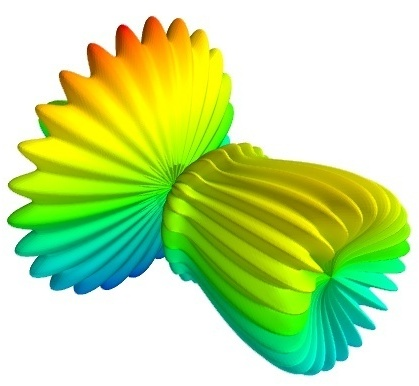The visualization is created by the single function mesh() in
the above.
Several examples of this kind are provided with mlab (see test_contour3d, test_points3d, test_plot3d_anim etc.). The above demo is available as test_mesh.
Under IPython these may be found by tab completing on mlab.test. You can also inspect the source in IPython via the handy mlab.test_contour3d??.

3D Plotting functions for numpy arrays
Visualization can be created in mlab by a set of functions operating on numpy arrays.
The mlab plotting functions take numpy arrays as input, describing the x, y,
and z coordinates of the data. They build full-blown visualizations: they create the data source, filters if necessary,
and add the visualization modules. Their behavior, and thus the visualization created, can be fine-tuned through keyword arguments, similarly to pylab. In addition, they all return the visualization module created, thus visualization can also be modified by
changing the attributes of this module.

Note

In this section, we only list the different functions. Each function is described in detail in the MLab

0D and 1D data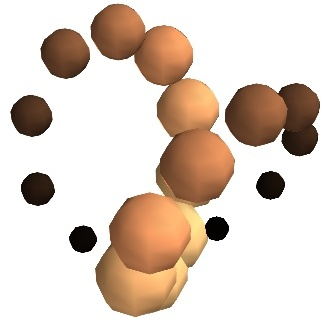points3d()
Plots glyphs (like points) at the position of the supplied data, described by x, y, z numpy
arrays of the same shape.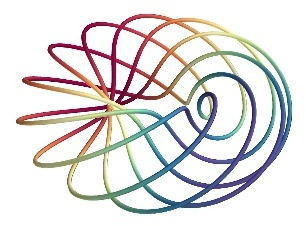plot3d()
Plots line between the supplied data, described by x, y, z 1D
numpy arrays of the same length.

2D dataimshow()
View a 2D array as an image.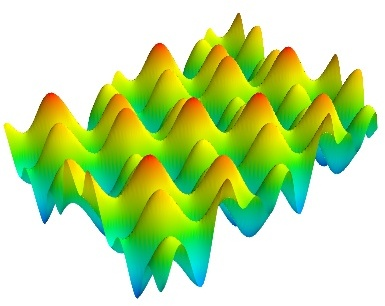surf()
View a 2D array as a carpet plot, with the z axis representation through elevation the value of the array points.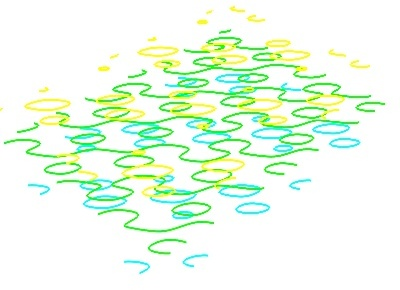contour_surf()
View a 2D array as line contours, elevated according to the value of the array points.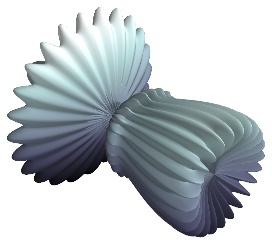mesh()
Plot a surface described by three 2D arrays, x, y, z giving
the coordinates of the data points as a grid.
Unlike surf(),
the surface is defined by its x, y and z coordinates
with no privileged direction. More complex surfaces can be created.barchart()
Plot an array s, or a set of points with explicit coordinates arrays, x, y and z,
as a bar chart, eg for histograms.
This function is very versatile and will accept 2D or 3D arrays, but also clouds of points, to position the bars.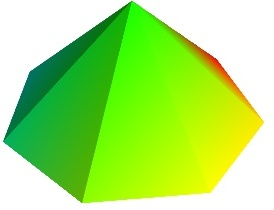triangular_mesh()
Plot a triangular mesh, fully specified by x, y and z coordinates
of its vertices, and the (n, 3) array of the indices of the triangles.

Vertical scale of surf() and contour_surf()
surf() and contour_surf() can
be used as 3D representation of 2D data. By default the z-axis is supposed to be in the same units as the x and y axis, but it can be auto-scaled to give a 2/3 aspect ratio. This behavior can be controlled by specifying the “warp_scale=’auto’”.

From data points to surfaces.
Knowing the positions of data points is not enough to define a surface, connectivity information is also required. With the functions surf() and mesh(),
this connectivity information is implicitly extracted from the shape of the input arrays: neighboring data points in the 2D input arrays are connected, and the data lies on a grid. With the function triangular_mesh(),
connectivity is explicitly specified. Quite often, the connectivity is not regular, but is not known in advance either. The data points lie on a surface, and we want to plot the surface implicitly defined. The delaunay2d filter does the required
nearest-neighbor matching, and interpolation, as shown in the (Surface
from irregular data example).

3D datacontour3d()
Plot iso-surfaces of volumetric data defined as a 3D array.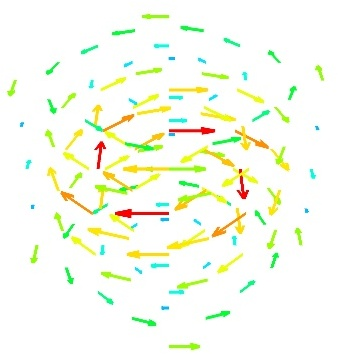quiver3d()
Plot arrows to represent vectors at data points. The x, y, z position
are specified by numpy arrays, as well as the u, v, w components
of the vectors.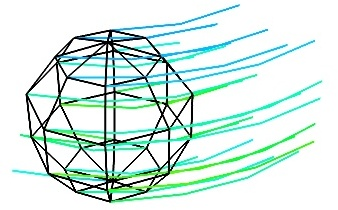flow()
Plot a trajectory of particles along a vector field described by three 3D arrays giving the u, v, wcomponents
on a grid.

Structured or unstructured data
contour3d() and flow() require
ordered data (to be able to interpolate between the points), whereas quiver3d() works
with any set of points. The required structure is detailed in the functions’ documentation.

Note

Many richer visualizations can be created by assembling data sources filters and modules. See the Assembling
pipelines with mlab and the Case studies of some visualizations sections.

Changing the looks of the visual objects created

Color:

The color of the objects created by a plotting function can be specified explicitly using the ‘color’ keyword argument of the function. This color is then applied uniformly to all the objects created.

If you want to vary the color across your visualization, you need to specify scalar information for each data point. Some functions try to guess this information: these scalars default to the norm of the vectors, for functions with vectors, or to the z elevation
for functions where it is meaningful, such as surf() or barchart().

This scalar information is converted into colors using the colormap, or also called LUT, for Look Up Table. The list of possible colormaps is:

accent       flag          hot      pubu     set2
autumn       gist_earth    hsv      pubugn   set3
black-white  gist_gray     jet      puor     spectral
blue-red     gist_heat     oranges  purd     spring
blues        gist_ncar     orrd     purples  summer
bone         gist_rainbow  paired   rdbu     winter
brbg         gist_stern    pastel1  rdgy     ylgnbu
bugn         gist_yarg     pastel2  rdpu     ylgn
bupu         gnbu          pink     rdylbu   ylorbr
cool         gray          piyg     rdylgn   ylorrd
copper       greens        prgn     reds
dark2        greys         prism    set1

The easiest way to choose the colormap, most adapted to your visualization is to use the GUI (as described in the next paragraph). The dialog to set the colormap can be found in the Colors and legends node.

To use a custom-defined colormap, for the time being, you need to write specific code, as show in Custom
colormap example.

Size of the glyph:

The scalar information can also be displayed in many different ways. For instance it can be used to adjust the size of glyphs positioned at the data points.

A caveat: Clamping: relative or absolute scaling Given six points positionned on a line with interpoint spacing 1:

x = [1, 2, 3, 4, 5, 6]
y = [0, 0, 0, 0, 0, 0]
z = y

If we represent a scalar varying from 0.5 to 1 on this dataset:

s = [.5, .6, .7, .8, .9, 1]

We represent the dataset as spheres, using points3d(),
and the scalar is mapped to diameter of the spheres:

from mayavi import mlab
pts = mlab.points3d(x, y, z, s)

By default the diameter of the spheres is not ‘clamped’, in other words, the smallest value of the scalar data is represented as a null diameter, and the largest is proportional to inter-point distance. The scaling is only relative, as can be seen on the resulting
figure:This behavior gives visible points for all datasets, but may not be desired if the scalar represents the size of the glyphs in the same unit as the positions specified.

In this case, you shoud turn auto-scaling off by specifying the desired scale factor:

pts = mlab.points3d(x, y, z, s, scale_factor=1)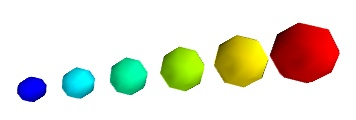Warning

In earlier versions of Mayavi (up to 3.1.0 included), the glyphs are not auto-scaled, and as a result the visualization can seem empty due to the glyphs being very small. In addition the minimum diameter of the glyphs is clamped to zero, and thus the glyph
are not scaled absolutely, unless you specify:

pts.glyph.glyph.clamping = False

More representations of the attached scalars or vectors:

There are many more ways to represent the scalar or vector information attached to the data. For instance, scalar data can be ‘warped’ into a displacement, e.g. using a WarpScalar filter, or the norm of scalar data can be extracted to a scalar
component that can be visualized using iso-surfaces with the ExtractVectorNorm filter.

Displaying more than one quantity:

You may want to display color related to one scalar quantity while using a second for the iso-contours, or the elevation. This is possible but requires a bit of work: see Atomic
orbital example.

If you simply want to display points with a size given by one quantity, and a color by a second, you can use a simple trick: add the size information using the norm of vectors, add the color information using scalars, create a quiver3d() plot
choosing the glyphs to symmetric glyphs, and use scalars to represent the color:

x, y, z, s, c = np.random.random((5, 10))
pts = mlab.quiver3d(x, y, z, s, s, s, scalars=c, mode='sphere')
pts.glyph.color_mode = 'color_by_scalar'
# Finally, center the glyphs on the data point
pts.glyph.glyph_source.glyph_source.center = [0, 0, 0]

Changing the scale and position of objects
Each mlab function takes an extent keyword argument, that allows to set its (x, y, z) extents. This give both control on the scaling in the different directions and the displacement
of the center. Beware that when you are using this functionality, it can be useful to pass the same extents to other modules visualizing the same data. If you don’t, they will not share the same displacement and scale.
The surf(), contour_surf(),
and barchart() functions,
which display 2D arrays by converting the values in height, also take a warp_scaleparameter, to control the vertical scaling.

Changing object properties interactively
Mayavi, and thus mlab, allows you to interactively modify your visualization.
The Mayavi pipeline tree can be displayed by clicking on the mayavi icon in the figure’s toolbar, or by using show_pipeline() mlab
command. One can now change the visualization using this dialog by double-clicking on each object to edit its properties, as described in other parts of this manual, or add new modules or filters by using this icons on the pipeline, or through the right-click
menus on the objects in the pipeline.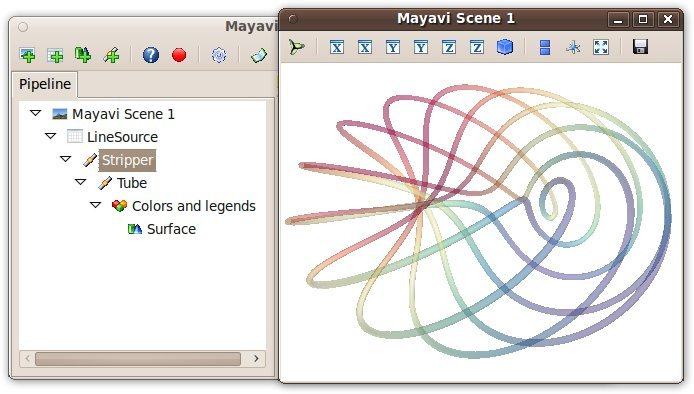The record feature
A very useful feature of this dialog can be found by pressing the red round button of the toolbar of the pipeline view. This opens up a recorder that tracks the changes made interactively
to the visualization via the dialogs, and generates valid lines of Python code. To find out about navigating through a program in the pipeline, see Organisation
of Mayavi visualizations: the pipeline.

In addition, for every object returned by a mlab function, this_object.edit_traits() brings
up a dialog that can be used to interactively edit the object’s properties. If the dialog doesn’t show up when you enter this command, please see Running
mlab scripts.

Using mlab with the full Envisage UI
Sometimes it is convenient to write an mlab script but still use the full envisage application so you can click on the menus and use other modules etc. To do this you may do the following before
you create an mlab figure:

from mayavi import mlab
mlab.options.backend = 'envisage'
f = mlab.figure()
# ...

This will give you the full-fledged UI instead of the default simple window.

Figures, legends, camera and decorations

Handling several figures
All mlab functions operate on the current scene, that we also call figure(),
for compatibility with matlab and pylab. The different figures are indexed by key that can be an integer or a string. A call to the figure() function
giving a key will either return the corresponding figure, if it exists, or create a new one. The current figure can be retrieved with the gcf() function.
It can be refreshed using the draw() function,
saved to a picture file using savefig() and
cleared using clf().

Figure decorations
Axes can be added around a visualization object with the axes() function,
and the labels can be set using the xlabel(), ylabel() andzlabel() functions.
Similarly, outline() creates
an outline around an object. title() adds
a title to the figure.
Color bars can be used to reflect the color maps used to display values (LUT, or lookup tables, in VTK parlance). colorbar() creates
a color bar for the last object created, trying to guess whether to use the vector data or the scalar data color maps. The scalarbar()and vectorbar() function
scan be used to create color bars specifically for scalar or vector data.
A small xyz triad can be added to the figure using orientation_axes().

Warning

The orientation_axes() was
named orientationaxes before release 3.2.

Moving the camera
The position and direction of the camera can be set using the view() function.
They are described in terms of Euler angles and distance to a focal point. The view() function
tries to guess the right roll angle of the camera for a pleasing view, but it sometimes fails. The roll() explicitly
sets the roll angle of the camera (this can be achieve interactively in the scene by pressing down the control key, while dragging the mouse, see Interaction
with the scene).
The view() and roll() functions
return the current values of the different angles and distances they take as arguments. As a result, the view point obtained interactively can be stored and reset using:

# Store the information
view = mlab.view()
roll = mlab.roll()

# Reposition the camera
mlab.view(*view)
mlab.roll(roll)

Rotating the camera around itself

You can also rotate the camera around itself using the roll, yaw and pitch methods of the camera object. This moves the focal point:

f = mlab.gcf()
camera = f.scene.camera
camera.yaw(45)

Unlike the view() and roll() function,
the angles are incremental, and not absolute.

Modifying zoom and view angle
The camera is entirely defined by its position, its focal point, and its view angle (attributes ‘position’, ‘focal_point’, ‘view_angle’). The camera method ‘zoom’ changes the view angle incrementally
by the specify ratio, where as the method ‘dolly’ translates the camera along its axis while keeping the focal point constant. The move() function
can also be useful in these regards.

Note

Camera parallel scale

In addition to the information returned and set by mlab.view and mlab.roll, a last parameter is needed to fully define the view point: the parallel scale of the camera, that control its view angle. It can be read (or set) with the following code:

f = mlab.gcf()
camera = f.scene.camera
cam.parallel_scale = 9

Running mlab scripts
Mlab, like the rest of Mayavi, is an interactive application. If you are not already in an interactive environment (see next paragraph), to interact with the figures or the rest of the drawing
elements, you need to use the show() function.
For instance, if you are writing a script, you need to call show() each
time you want to display one or more figures and allow the user to interact with them.

Using mlab interactively
Using IPython, mlab instructions can be run interactively,
or in scripts using IPython‘s %run command:

In : %run my_script

You need to start IPython with the -wthread option,
that became –gui=wx in the recent IPython versions (when installed with EPD, thepylab start-menu
link does this for you). In this environment, the plotting commands are interactive: they have an immediate effect on the figure, alleviating the need to use the show() function.
Mlab can also be used interactively in the Python shell of the mayavi2 application, or in any interactive Python shell of wxPython-based application (such as other Envisage-based applications,
or SPE, Stani’s Python Editor).

Using together with Matplotlib’s pylab
If you want to use Matplotlib’s pylab with Mayavi’s mlab in IPython you should:

if your IPython version is greater than 0.11: start IPython with:

$ipython --gui=wx --pylab=wx else, if your IPython version is greater than 0.8.4: start IPython with the following options:$ ipython -pylab -wthread

elsewhere, start IPython with the -wthread option:

and before importing pylab, enter the following Python commands:

>>> import matplotlib
>>> matplotlib.use('WxAgg')
>>> matplotlib.interactive(True)

If you want matplotlib and mlab to work together by default in IPython, you can change you default matplotlib backend, by editing the~/.matplotlib/matplotlibrc to add the following
line:

backend     : WXAgg

Capturing mlab plots to integrate in pylab
Starting from Mayavi version 3.4.0, the mlab screenshot() can
be used to take a screenshot of the current figure, to integrate in a matplotlib plot.

In scripts
Mlab commands can be written to a file, to form a script. This script can be loaded in the Mayavi application using the File->Open file menu entry, and executed using the File->Refresh
code menu entry or by pressing Control-r. It can also be executed during the start of the Mayavi application using
the -x command line switch.
As mentioned above, when running outside of an interactive environment, for instance with python myscript.py, you need to call theshow() function
(as shown in the demo above) to pause your script and have the user interact with the figure.
You can also use show() to
decorate a function, and have it run in the event-loop, which gives you more flexibility:

from mayavi import mlab
from numpy import random

@mlab.show
def image():
mlab.imshow(random.random((10, 10)))

With this decorator, each time the image function is called, mlab makes sure an interactive environment is running before executing the image function. If
an interactive environment is not running, mlab will start one and the image function will not return until it is closed.

Animating the data
Often it isn’t sufficient to just plot the data. You may also want to change the data of the plot and update the plot without having to recreate the entire visualization, for instance to do animations,
or in an interactive application. Indeed, recreating the entire visualization is very inefficient and leads to very jerky looking animations. To do this, mlab provides a very convenient way to change the data of an existing mlab visualization. Consider a very
simple example. The mlab.test_simple_surf_anim function has this code:

import numpy as np
from mayavi import mlab
x, y = np.mgrid[0:3:1,0:3:1]
s = mlab.surf(x, y, np.asarray(x*0.1, 'd'))

for i in range(10):
s.mlab_source.scalars = np.asarray(x*0.1*(i+1), 'd')

The first two lines define a simple plane and view that. The next three lines animate that data by changing the scalars producing a plane that rotates about the origin. The key here is that the s object
above has a special attribute called mlab_source. This sub-object allows us to manipulate the points and scalars. If we wanted to change the x values we could set that too by:

s.mlab_source.x = new_x

The only thing to keep in mind here is that the shape of x should not be changed.
If multiple values have to be changed, you can use the set method of the mlab_source to set them as shown in the more complicated example below:

# Produce some nice data.
n_mer, n_long = 6, 11
pi = np.pi
dphi = pi/1000.0
phi = np.arange(0.0, 2*pi + 0.5*dphi, dphi, 'd')
mu = phi*n_mer
x = np.cos(mu)*(1+np.cos(n_long*mu/n_mer)*0.5)
y = np.sin(mu)*(1+np.cos(n_long*mu/n_mer)*0.5)
z = np.sin(n_long*mu/n_mer)*0.5

# View it.
l = plot3d(x, y, z, np.sin(mu), tube_radius=0.025, colormap='Spectral')

# Now animate the data.
ms = l.mlab_source
for i in range(10):
x = np.cos(mu)*(1+np.cos(n_long*mu/n_mer +
np.pi*(i+1)/5.)*0.5)
scalars = np.sin(mu + np.pi*(i+1)/5)
ms.set(x=x, scalars=scalars)

Notice the use of the set method above. With this method, the visualization is recomputed only once. In this case, the shape of the new arrays has not changed, only their values have.
If the shape of the array changes then one should use the reset method as shown below:

x, y = np.mgrid[0:3:1,0:3:1]
s = mlab.surf(x, y, np.asarray(x*0.1, 'd'),
representation='wireframe')
# Animate the data.
fig = mlab.gcf()
ms = s.mlab_source
for i in range(5):
x, y = np.mgrid[0:3:1.0/(i+2),0:3:1.0/(i+2)]
sc = np.asarray(x*x*0.05*(i+1), 'd')
ms.reset(x=x, y=y, scalars=sc)
fig.scene.reset_zoom()

Many standard examples for animating data are provided with mlab. Try the examples with the name mlab.test_<name>_anim, i.e. where the name ends with an _anim to see how
these work and run.

Note

It is important to remember distinction between set and reset. Use set or directly set the attributes (x, y, scalars etc.) when you are not changing the shape of the data but only
the values. Use reset when the arrays are changing shape and size. Reset usually regenerates all the data and can be inefficient when compared to set or directly setting the traits.

Warning

When creating a Mayavi pipeline, as explained in the following subsection, instead of using ready-made plotting function, the mlab_source attribute is created only on sources created via mlab. Pipeline created entirely using mlab will
present this attribute.

Note

If you are animating several plot objects, each time you modify the data with there mlab_source attribute, Mayavi will trigger a refresh of the scene. This operation might take time, and thus slow your animation. In this case, the tip Accelerating
a Mayavi script may come in handy.

Assembling pipelines with mlab
The plotting functions reviewed above explore only a small fraction of the visualization possibilities of Mayavi. The full power of Mayavi can only be unleashed through the control of the pipeline
itself. As described in the An overview of Mayavi section, a visualization
in Mayavi is created by loading the data in Mayavi with data source object, optionally transforming the data throughFilters,
and visualizing it with Modules. The mlab functions build complex pipelines for
you in one function, making the right choice of sources, filters, and modules, but they cannot explore all the possible combinations.
Mlab provides a sub-module pipeline which contains functions to populate the pipeline easily from scripts. This module is accessible in mlab: mlab.pipeline,
or can be imported from mayavi.tools.pipeline.
When using an mlab plotting function, a pipeline is created: first a source is created from numpy arrays, then modules, and possibly filters, are added. The resulting
pipeline can be seen for instance with the mlab.show_pipeline command. This information can be used to create the very same pipeline directly using the pipeline scripting module, as the names of the functions required to create each
step of the pipeline are directly linked to the default names of the objects created by mlab on the pipeline. As an example, let us create a visualization using surf():

import numpy as np
a = np.random.random((4, 4))
from mayavi import mlab
mlab.surf(a)
mlab.show_pipeline()

The following pipeline is created:

Array2DSource
\__ WarpScalar
\__ PolyDataNormals
\__ Colors and legends
\__ Surface

The same pipeline can be created using the following code:

src = mlab.pipeline.array2d_source(a)
warp = mlab.pipeline.warp_scalar(src)
normals = mlab.pipeline.poly_data_normals(warp)
surf = mlab.pipeline.surface(normals)

Data sources
The mlab.pipeline module contains functions for creating various data sources from arrays. They are fully documented in details in theMlab
pipeline-control reference. We give a small summary of the possibilities here.
Mayavi distinguishes sources with scalar data, and sources with vector data, but more important, it has different functions to create sets of unconnected
points, with data attached to them, or connected data points describing continuously varying quantities that can be interpolated between data points, often called fields in physics or engineering.

Unconnected sources:

scalar_scatter() (creates
a PolyData)vector_scatter() (creates
an PolyData)implicitly-connected sources:

scalar_field() (creates
an ImageData)vector_field() (creates
an ImageData)array2d_source() (creates
an ImageData)Explicitly-connected sources:

line_source() (creates
an PolyData)triangular_mesh_source() (creates
an PolyData)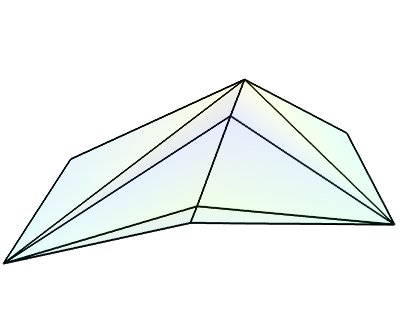All the mlab.pipline source factories are functions that take numpy arrays and return the Mayavi source object that was added to the pipeline. However, the implicitly-connected sources
require well-shaped arrays as arguments: the data is supposed to lie on a regular, orthogonal, grid of the same shape as the shape of the input array, in other words, the array describes an image, possibly 3 dimensional.

Note

More complicated data structures can be created, such as irregular grids or non-orthogonal grid. See the
section on data structures.

Modules and filters
For each Mayavi module or filter (see Modules and Filters),
there is a corresponding mlab.pipeline function. The name of this function is created by replacing the alternating capitals in the module or filter name by underscores. Thus ScalarCutPlane corresponds toscalar_cut_plane.
In general, the mlab.pipeline module and filter factory functions simply create and connect the corresponding object. However they can also contain addition logic, exposed as keyword
arguments. For instance they allow to set up easily a colormap, or to specify the color of the module, when relevant. In accordance with the goal of the mlab interface to make frequent operations simple, they use the keyword arguments to choose
the properties of the created object to suit the requirements. It can be thus easier to use the keyword arguments, when available, than to set the attributes of the objects created. For more information, please check out the docstrings. Full, detailed, usage
examples are given in the next subsection.

Case studies of some visualizations

Visualizing volumetric scalar data
There are three main ways of visualizing a 3D scalar field. Given the following field:

import numpy as np
x, y, z = np.ogrid[-10:10:20j, -10:10:20j, -10:10:20j]
s = np.sin(x*y*z)/(x*y*z)

Iso-Surfaces:

To display iso surfaces of the field, the simplest solution is simply to use the mlab contour3d() function:

mlab.contour3d(s)The problem with this method is that the outer iso-surfaces tend to hide inner ones. As a result, quite often only one iso-surface can be visible.

Volume rendering:

Volume rendering is an advanced technique in which each voxel is given a partly transparent color. This can be achieved with mlab.pipeline using the scalar_field() source,
and the volume module:

mlab.pipeline.volume(mlab.pipeline.scalar_field(s))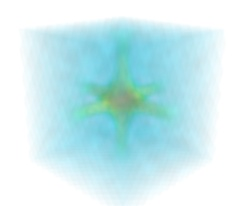For such a visualization, tweaking the opacity transfer function is critical to achieve a good effect. Typically, it can be useful to limit the lower and upper values to the 20 and 80 percentiles of the data, in order to have a reasonable fraction of the volume
transparent:

mlab.pipeline.volume(mlab.pipeline.scalar_field(s), vmin=0, vmax=0.8)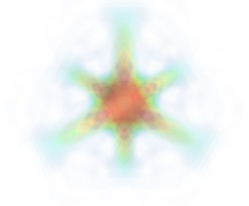It is useful to open the module’s dialog (eg through the pipeline interface, or using it’s edit_traits() method) and tweak the color transfer function to render the transparent low-intensity regions of the image. For this module, the LUT
as defined in the Colors and legends node are not used

The limitations of volume rendering is that, while it is often very pretty, it can be difficult to analyze the details of the field with it.

Cut planes:

While less impressive, cut planes are a very informative way of visualizing the details of a scalar field:

mlab.pipeline.image_plane_widget(mlab.pipeline.scalar_field(s),
plane_orientation='x_axes',
slice_index=10,
)
mlab.pipeline.image_plane_widget(mlab.pipeline.scalar_field(s),
plane_orientation='y_axes',
slice_index=10,
)
mlab.outline()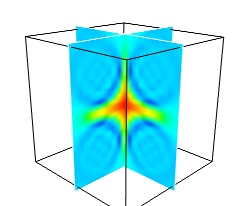The image plane widget can only be used on regular-spaced data, as created by mlab.pipeline.scalar_field, but it is very fast. It should thus be prefered to the scalar cut plane, when possible.

Clicking and dragging the cut plane is an excellent way of exploring the field.

A combination of techniques:

Finally, it can be interesting to combine cut planes with iso-surfaces and thresholding to give a view of the peak areas using the iso-surfaces, visualize the details of the field with the cut plane, and the global mass with a large iso-surface:

src = mlab.pipeline.scalar_field(s)
mlab.pipeline.iso_surface(src, contours=[s.min()+0.1*s.ptp(), ], opacity=0.1)
mlab.pipeline.iso_surface(src, contours=[s.max()-0.1*s.ptp(), ],)
mlab.pipeline.image_plane_widget(src,
plane_orientation='z_axes',
slice_index=10,
)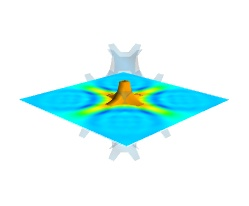In some cases, though not in our example, it might be usable to insert a threshold filter before the cut plane, eg:to remove area with values below ‘s.min()+0.1*s.ptp()’. In this case, the cut plane needs to be implemented withmlab.pipeline.scalar_cut_plane as
the data looses its structure after thresholding.

Visualizing a vector field
A vector field, i.e., vectors continuously defined in a volume, can be difficult to visualize, as it contains a lot of information. Let us explore different visualizations for the velocity field
of a multi-axis convection cell , in hydrodynamics, as defined by its components sampled
on a grid, u, v, w:

import numpy as np
x, y, z = np.mgrid[0:1:20j, 0:1:20j, 0:1:20j]

u =    np.sin(np.pi*x) * np.cos(np.pi*z)
v = -2*np.sin(np.pi*y) * np.cos(2*np.pi*z)
w = np.cos(np.pi*x)*np.sin(np.pi*z) + np.cos(np.pi*y)*np.sin(2*np.pi*z)

Quiver:

The simplest visualization of a set of vectors, is using the mlab function quiver3d:

mlab.quiver3d(u, v, w)
mlab.outline()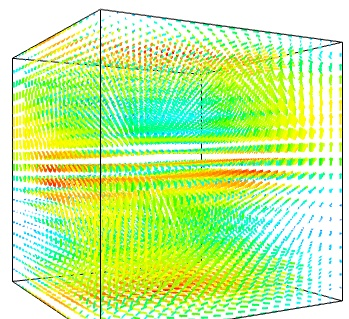The main limitation of this visualization is that it positions an arrow for each sampling point on the grid. As a result the visualization is very busy.

We can use the fact that we are visualizing a vector field, and not just a bunch of vectors, to reduce the amount of arrows displayed. For this we need to build a vector_field source, and apply to it the vectors module, with some masking
parameters (here we keep only one point out of 20):

src = mlab.pipeline.vector_field(u, v, w)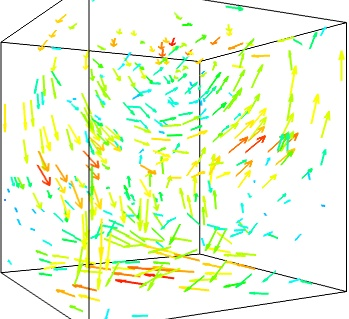A cut plane:

If we are interested in displaying the vectors along a cut, we can use a cut plane. In particular, we can inspect interactively the vector field by moving the cut plane along: clicking on it and dragging it can give a very clear understanding of the vector
field: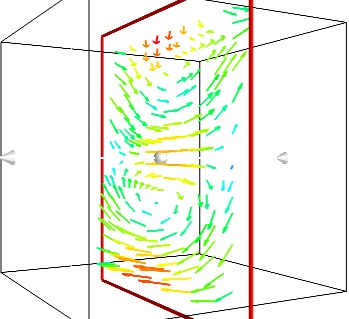Iso-Surfaces of the magnitude:

An important parameter of the vector field is its magnitude. It can be interesting to display iso-surfaces of the normal of the vectors. For this we can create a scalar field from the vector field using the ExtractVectorNorm filter, and use the Iso-Surface
module on it. When working interactively, a good understanding of the magnitude of the field can be gained by changing the values of the contours in the object’s property dialog.

magnitude = mlab.pipeline.extract_vector_norm(src)
mlab.pipeline.iso_surface(magnitude, contours=[1.9, 0.5])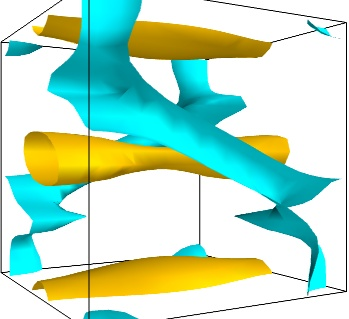The Flow, or the field lines:

For certain vector fields, the line of flow along the field can have an interesting meaning. For instance this can be interpreted as a trajectory in hydrodynamics, or field lines in electro-magnetism. We can display the flow lines originating for a certain
seed surface using the streamline module, or the mlab flow() function,
which relies on streamlineinternally:

flow = mlab.flow(u, v, w, seed_scale=1,
seed_resolution=5,
integration_direction='both')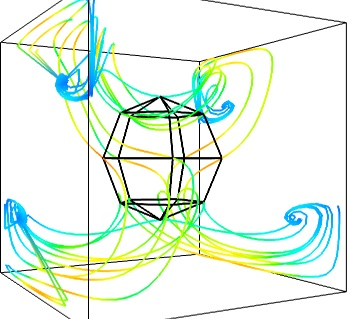A combination of techniques:

Giving a meaningful visualization of a vector field is a hard task, and one must use all the tools at hand to illustrate his purposes. It is important to choose the message conveyed. No one visualization will tell all about a vector field. Here is an example
of a visualization made by combining the different tools above:

mlab.figure(fgcolor=(0., 0., 0.), bgcolor=(1, 1, 1))
src = mlab.pipeline.vector_field(u, v, w)
magnitude = mlab.pipeline.extract_vector_norm(src)

# We apply the following modules on the magnitude object, in order to
# be able to display the norm of the vectors, eg as the color.
iso = mlab.pipeline.iso_surface(magnitude, contours=[1.9, ], opacity=0.3)

line_width=1,
color=(.8, .8, .8),
scale_factor=4.)

flow = mlab.pipeline.streamline(magnitude, seedtype='plane',
seed_visible=False,
seed_scale=0.5,
seed_resolution=1,
linetype='ribbon',)

scale_factor=4,
colormap='jet',
plane_orientation='x_axes')Note

Although most of this section has been centered on snippets of code to create visualization objects, it is important to remember that Mayavi is an interactive program, and that the properties of these objects can be modified interactively, as described in Changing
object properties interactively. It is often impossible to choose the best parameters for a visualization before hand. Colors, contour values, colormap, view angle, etc... should be chosen interactively. If reproducibiles are required, the chosen
values can be added in the original script.

Moreover, the mlab functions expose only a small fraction of the possibilities of the visualization objects. The dialogs expose more of these functionalities, that are entirely controlled by the attributes of the objects returned by the mlab functions.
These objects are very rich, as they are built from VTK objects. It can be hard to find the right attribute to modify when exploring them, or in the VTK documentation, thus the easiest way is to modify them interactively using the pipeline view dialog and
use the record feature to find out the corresponding
lines of code. See Organisation of Mayavi visualizations: the
pipeline to understand better the link between the lines of code generated by the record feature and mlab. .


Toussaint, V.; Carriere, P. & Raynal, F. A numerical Eulerian approach to mixing by chaotic advection Phys. Fluids, 1995, 7, 2587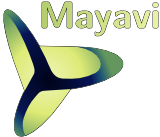mlab:
Python scripting for 3D plotting

A demo3D Plotting functions for numpy arrays

0D and 1D data2D data3D data
Changing the looks of the visual objects created

Adding color or size variationsChanging the scale and position of objectsChanging object properties interactively
Figures, legends, camera and decorations

Handling several figuresFigure decorationsMoving the camera
Running mlab scripts

Using mlab interactivelyUsing together with Matplotlib’s pylabIn scripts
Animating the dataAssembling pipelines with mlab

Data sourcesModules and filters
Case studies of some visualizations

Visualizing volumetric scalar dataVisualizing a vector field

Previous topic
Filters

Next topic

Show Source

Quick search

Enter search terms or a module, class or function name.

only search Mayavi
documentation

Citing Mayavi
If you publish articles using Mayavi, please cite Mayavi.
We need these citations to justify time and resources on the software.

indexnext |previous |mayavi 4.3.1 documentation »

© Copyright 2008-2014, Prabhu Ramachandran, Gaël Varoquaux. Last updated on Jan 30, 2014. Created using Sphinx 1.1.2.


展开全文• 用过mayavi的人，都知道mayavi的好，作图非常的漂亮，三维图像非常的逼真，但是由于官方似乎还不直接支持python3（也没有说不支持），所以用pip和conda都无法安装，只好下载编译文件，自行处理各种依赖关系，本博文...
更新时间：2019-9-9
python3已经完全支持mayavi的依赖了，大家直接安装。
 pip install mayavi
pip install PyQt5

用过mayavi的人，都知道mayavi的好，作图非常的漂亮，三维图像非常的逼真，但是由于官方似乎还不直接支持python3（也没有说不支持），所以用pip和conda都无法安装，只好下载编译文件，自行处理各种依赖关系，本博文详细介绍了如何去安装各种依赖包，博主安装的mayavi-4.5.0版本。
** python2 的朋友直接使用 conda install mayavi 即可 **
1 包的安装顺序
首先给出各种包的下载地址，已打包好，下载解压即可：链接：http://pan.baidu.com/s/1dER3XWt 密码：6mrl
** 如果链接失效，联系博主重新上传 **
1.1 安装顺序：

apptools-4.4.0, configobj-5.0.6, pyface-5.1.0, traitsui-5.1.0
这四个包都是库源文件，所以到各自文件夹中打开cmd，运行： python setup.py install, 例如安装apptools，在apptools文件夹中打开cmd，输入：python setup.py install， 之后等待安装成功。依次安装上述4个库

安装pyqt4，traits，VTK,mayavi
这四个由于是编译后的二进制文件，直接在该目录进行安装即可。在该目录打开cmd，输入：pip install 库名称。例如安装pyqt4：pip install PyQt4-4.11.4-cp36-cp36m-win_amd64.whl

依次安装上述库文件
1.2 注意事项： 我打包的文件都适用py3.6版本的，其他版本自行百度。
2 测试
import numpy as np
from mayavi import mlab
x, y = np.ogrid[-2:2:20j, -2:2:20j]
z = x * np.exp( - x**2 - y**2)
pl = mlab.surf(x, y, z, warp_scale="auto")
mlab.axes(xlabel='x', ylabel='y', zlabel='z')
mlab.outline(pl)
mlab.show()

运行结果：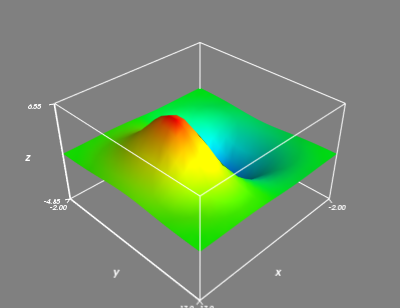敲黑板，如果各位博友遇到什么问题可以给我留言交流，一起解决


展开全文python3 VTK
• Mayavi 的参考手册，适合初学者和expert。 User guide: full table of contents An overview of Mayavi Introduction What is Mayavi2? Technical details Using Mayavi as an application, or a library? Scenes...
• Mayavi, 3D visualization of scientific data. Part of the Enthought Tool Suite. Requires VTK.
• Mayavi是python的一个包，提供方便的可视化方案。目前（20150809）Mayavi还没有py3的支持，以下安装环境在python 2.7.10下进行 安装Mayavi： 1. 通过pip install mayavi自动检测并且安装大部分的依赖包： ...

Mayavi是python的一个包，提供方便的可视化方案。目前（20150809）Mayavi还没有py3的支持，以下安装环境在python 2.7.10下进行
安装Mayavi：
1. 通过pip install mayavi自动检测并且安装大部分的依赖包：
apptools、traits、traitsui、configobj、pyface、six
如果速度太慢可以参见之前随笔更改pip源为豆瓣源：pip install -i http://pypi.douban.com/simple --trusted-host pypi.douban.com
2. 此时安装Mayavi仍然报错：ImportError: No module named vtk
需要安装vtk支持：

apt-get install vtk6
ubuntu 下使用apt-get 可以直接安装vtk，可能需要安装的依赖会比较多，需要花费较长时间，可参考之前随笔更改ubuntu源为阿里源：http://www.cnblogs.com/lyon2014/p/4715379.html
安装python-vtk：
apt-get install python-vtk
3. 此时，如果你用的python就是系统的python，应该就有了vtk模块，测试：
python -c 'import vtk'
没有报错就已经成功了。到这里就可以直接跳过3、4两步。
但是如果你和我一样用的是自己安装的python，或者用pyenv管理python版本，此时仍然找不到vtk模块，直到执行pyenv global system切换回系统python才能找到vtk模块
4. 安装vtk模块
vtk官网死活打不开，重新安装vtk遇到困难，但是没关系，还有另外的解决方案：将系统安装的vtk添加到自己的python中
首先找到系统安装的python-vtk路径，如ubuntu下，apt-get会默认安装在/usr/share/pyshared/以及相应编译的库包安装在：/usr/lib/pyshared/python2.7/vtk中，通过查看/usr/share/python-support/python-vtk.public文件可以看到相关信息
第一种方案是将这两个目录添加到PYTHONPATH环境变量中，个人比较反感污染PYTHONPATH变量，这会导致使用其他版本python的时候难以控制；
第二种方案是将/usr/share/pyshared/vtk 链接到当前python的lib目录下，并且把/usr/lib/pyshared/python2.7/vtk中的内容也链接过来：

1 cd ~/.pyenv/versions/2.7.10/lib/python2.7/site-packages
2 mkdir vtk
3 ln -s /usr/share/pyshared/vtk/* ./
4 ln -s /usr/lib/pyshared/python2.7/vtk/* ./

5. 更改python的unicode支持（建议先看完第6点再操作）
如果你和我一样使用pyenv，则默认编译安装的python支持的unicode是ucs2的，此时：
python -c 'import vtk'
则会报错：ImportError: /usr/lib/libvtkPythonCore.so.5.8: undefined symbol: _PyUnicodeUCS4_AsDefaultEncodedString
需要重新编译，使其支持UCS4
首先重新下载python：pyenv install -k 2.7.10
等待下载完成，开始安装的时候，取消，然后进入下载目录：
cd ~/.pyenv/sources/2.7.10
tar zxvf Python-2.7.10.tgz
cd Python-2.7.10/
./configure --prefix=/home/vagrant/.pyenv/versions/2.7.10 --enable-unicode=ucs4
上面prefix目录填你的pyenv对应的版本安装目录，使用绝对路径，一般来说是: /home/用户名/.pyenv/versions/版本号，只有配置为这个目录才能使pyenv调用的python为这次编译安装的python；如果你没有用pyenv，忽略此条；
make && make install
等待安装完成
测试：python -c 'import vtk'
没有报错，安装成功
6. 注意：一旦你完成第五步，意味着之前安装的包如果使用了ucs2编码，则会找不到关于ucs2的内容（比如numpy），这个时候需要卸载重装相应的包，所以关于unicode是很坑的一个地方，需要检查并且重新编译各种包，比较好的办法是，不要去更改原来的python，使用pyenv新安装一个python，并且编译支持ucs4，之后将可能用到的包一一安装；
7. 当你搞定所有的问题之后，终于可以安装mayavi了：
pip install mayavi
大功告成
出于学习的需要，会学习mayavi，但是我是一万个不情愿使用更新滞后的包的，在其支持py3之前应该不会使用，可能会被其他包替代吧。
继：
如果使用mayavi的话，最好还是切换到系统python，否则会有很多的依赖问题；
安装好mayavi之后，如果需要使用，则需要安装enthghout公司的其他包：VTK, appinst, apptools, envisage, traitsui, traits, pyface, configobj
这些包都可以通过pip快速安装，安装好之后，还需要依赖的界面工具，可以是wxPython或者是PyQT中的一种，否则无法成功使用traits包；
以PyQT为例，在安装PyQT之前，首先确认QT已经成功安装，然后再安装PyQT，这里比较郁闷的是pip上的pyqt好像都装不了，使用homebrew安装pyqt时发现依赖sip

转载于:https://www.cnblogs.com/lyon2014/p/4716099.html
展开全文• mayavi 4.6.2 安装包及依赖包 主要有依赖包 PyQt traits VTK mayavi 附带下载说明 注意自己的python版本
• ## Mayavi 入门

千次阅读 2018-01-06 16:34:20
Mayavi 入门 Mayavi 库基本元素 Mayavi.mlab Mayavi API 绘图实例 from mayavi import mlab x = [[-1, 1, 1, -1, -1], [-1, 1, 1, -1, -1]] y = [[-1, -1, -1, -1, -1], [1, 1, 1
• Mayavi库的基本元素Mayavi库中主要有两大部分功能　一类是用于处理图形可视化和图形操作的mlab模块　一类是操作管线对象窗口对象的apimlab包含 　绘图函数：实现已有的数据进行可视化显示，可以是numpy数组构建的，...
• ## python mayavi三维绘图

万次阅读 多人点赞 2017-08-02 19:22:51
网上下载mayavi的官方帮助文档，里面有很多例子，下面的记录都是查看手册后得到的。 http://code.enthought.com/projects/mayavi/docs/development/latex/mayavi/mayavi_user_guide.pdf python的mayavi.mlab库中的...python
• 参考 ...mayavi绘图基础 mayavi绘制进阶 多窗口 需要每个窗口建立时，生成scene，并生成figure，获取figure句柄。才能每个窗口独立操作。 参考代码在安装路径：/home/xxx/.local/lib/python...
• mayavi安装依赖包，包含mayavi安装包，基于python3.6.0，包含anaconda3-4.3.1安装包
• 摘要：Mayavi科学数据3维可视化器 开发： ： 说明文件： : 当前构建状态 蔚蓝 变体 状态 linux_64_c_compiler_version7fortran_compiler_version7numpy1.16python3.6 .____ cpython linux_64_c_compiler_...Shell
• ## Python MayaVi

千次阅读 2014-07-24 15:07:42
http://www.sam.math.ethz.ch/~raoulb/teaching/PythonTutorial/tips_mayavi2.html ...The enthought.mayavi.mlab module, that we call mlab, provides an easy way to visualize data ipython
• C:\Users\Administrator\AppData\Roaming\Python\Python39\site-packages\mayavi\tools\engine_manager.py", line 13, in <module>  from mayavi.core.registry import registry  File "...python 有问必答
• Mayavi基于Python作为VTK的载体在三维图像的渲染和交互操作方面具有很多优势。最近分析数据的混沌的状态时需要在四维层面上表现数据的效果。首先在matlab，tecplot和origin试验了一番。可以说他们都可以实现，但在...
• 安装教程 需要按顺序安装： qypt4 VTK traits Mayavi 安装包下载地址： https://www.lfd.uci.edu/~gohlke/pythonlibs/...